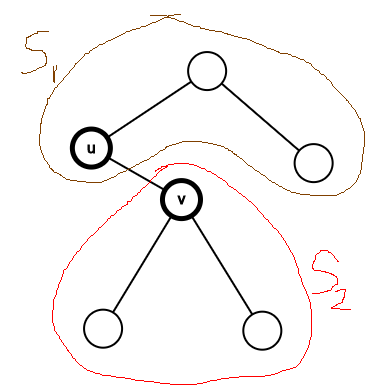Setter: Prasanna Kumar
Tester: Anshu Garg, Nishant Shah

EASY-MEDIUM

# PROBLEM:

Given a rooted tree with N nodes. Initially, all nodes have colour black. You are also given Q queries of the following form:

• Determine the number of paths passing through edge u\to v, with both endpoint nodes having the colour black.
• Invert the colour of all nodes in the subtree of node u.

# EXPLANATION:(Leonardo gave the world ‘Mona Lisa’; here’s my masterpiece)

Let b_1 and b_2 correspond to the number of black nodes in S_1 and S_2 (from above picture), respectively. For queries of type 1, the answer is equal to

b_1*b_2 \implies ((b_1+b_2)-b_2)*b_2

where b_1+b_2 is equivalent to the number of black nodes in the whole graph.

Thus we have reduced the problem to:

• Invert the color of all nodes in the subtree of u
• Determine the number of black nodes in the subtree of u (and subtree of node 1 - to find the total number of black nodes).

This can be solved efficiently using a Segment Tree (with lazy propagation) on the Euler Tour of the rooted tree.

How?

Run Euler Tour on the tree; let tin_u and tout_v be the entry and exit times of node u in the tour.

Consider an array A such that A_{tin_u} = 1 if node u is black. Initially, A_i = 1 for all valid i.

• For a flip operation (query type 2) on the subtree of u, invert the value of all elements of A in the range [tin_u, tout_u].
• To query the number of black nodes in the subtree of u, sum all elements of A in the range [tin_u, tout_u].

Both operations can be done in O(\log N) per query, using segment trees with lazy propagation.

# TIME COMPLEXITY:

Running Euler Tour takes O(N). Building the segment tree takes O(N\log N). Each query takes O(\log N). The total complexity is thus:

O(N+N\log N+Q\log N) \approx O((N+Q)\log N)

# SOLUTIONS:

Editorialist’s solution can be found here

Experimental: For evaluation purposes, please rate the editorial (1 being poor and 5 excellent)

• 1
• 2
• 3
• 4
• 5

0 voters

You mean bi*b2 = ((b1+b2)-b1)*b1

1 Like